Chapter 6. Gases

# Other Gas Laws

Learning Objectives

1. Review other simple gas laws.
2. Learn and apply the combined gas law.

You may notice in Boyle’s law and Charles’s law that we actually refer to four physical properties of a gas: pressure (P), volume (V), temperature (T), and amount (in moles, n). We do this because these are the only four independent physical properties of a gas. There are other physical properties, but they are all related to one (or more) of these four properties.

Boyle’s law is written in terms of two of these properties, with the other two being held constant. Charles’s law is written in terms of two different properties, with the other two being held constant. It may not be surprising to learn that there are other gas laws that relate other pairs of properties—as long as the other two are held constant. Here we will mention a few.

relates pressure with absolute temperature. In terms of two sets of data, Gay-Lussac’s law is: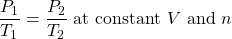Note that it has a structure very similar to that of Charles’s law, only with different variables—pressure instead of volume. introduces the last variable for amount. The original statement of Avogadro’s law states that equal volumes of different gases at the same temperature and pressure contain the same number of particles of gas. Because the number of particles is related to the number of moles (1 mol = 6.022 × 1023 particles), Avogadro’s law essentially states that equal volumes of different gases at the same temperature and pressure contain the same amount (moles, particles) of gas. Put mathematically into a gas law, Avogadro’s law is: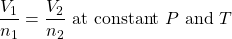(First announced in 1811, it was Avogadro’s proposal that volume is related to the number of particles that eventually led to naming the number of things in a mole as Avogadro’s number.) Avogadro’s law is useful because for the first time we are seeing amount, in terms of the number of moles, as a variable in a gas law.

Example 6.7

# Problem

A 2.45 L volume of gas contains 4.5 × 1021 gas particles. How many gas particles are there in 3.87 L if the gas is at constant pressure and temperature?

## Solution

We can set up Avogadro’s law as follows: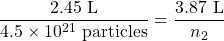We algebraically rearrange to solve for n2: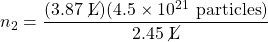The L units cancel, so we solve for n2:

n2 = 7.1 × 1021 particles

# Test Yourself

A 12.8 L volume of gas contains 3.00 × 1020 gas particles. At constant temperature and pressure, what volume do 8.22 × 1018 gas particles fill?

0.351 L

The variable n in Avogadro’s law can also stand for the number of moles of gas in addition to the number of particles.

One thing we notice about all the gas laws is that, collectively, volume and pressure are always in the numerator, and temperature is always in the denominator. This suggests that we can propose a gas law that combines pressure, volume, and temperature. This gas law is known as the , and its mathematical form is: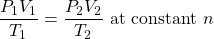This allows us to follow changes in all three major properties of a gas. Again, the usual warnings apply about how to solve for an unknown algebraically (isolate it on one side of the equation in the numerator), units (they must be the same for the two similar variables of each type), and units of temperature (must be in kelvins).

Example 6.8

# Problem

A sample of gas at an initial volume of 8.33 L, an initial pressure of 1.82 atm, and an initial temperature of 286 K simultaneously changes its temperature to 355 K and its volume to 5.72 L. What is the final pressure of the gas?

## Solution

We can use the combined gas law directly; all the units are consistent with each other, and the temperatures are given in Kelvin. Substituting,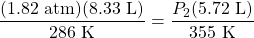We rearrange this to isolate the P2 variable all by itself. When we do so, certain units cancel: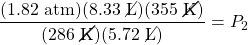Multiplying and dividing all the numbers, we get:

P2 = 3.29 atm

Ultimately, the pressure increased, which would have been difficult to predict because two properties of the gas were changing.

# Test Yourself

If P1 = 662 torr, V1 = 46.7 mL, T1 = 266 K, P2 = 409 torr, and T2 = 371 K, what is V2?

105 mL

As with other gas laws, if you need to determine the value of a variable in the denominator of the combined gas law, you can either cross-multiply all the terms or just take the reciprocal of the combined gas law. Remember, the variable you are solving for must be in the numerator and all by itself on one side of the equation.

Key Takeaways

• There are other gas laws that relate any two physical properties of a gas.
• The combined gas law relates pressure, volume, and temperature of a gas.

Exercises

# Questions

1. State Gay-Lussac’s law.
3. Use Gay-Lussac’s law to determine the final pressure of a gas whose initial pressure is 602 torr, initial temperature is 356 K, and final temperature is 277 K. Assume volume and amount are held constant.
4. Use Gay-Lussac’s law to determine the final temperature of a gas whose initial pressure is 1.88 atm, initial temperature is 76.3 K, and final pressure is 6.29 atm. Assume volume and amount are held constant.
5. If 3.45 × 1022 atoms of Ar have a volume of 1.55 L at a certain temperature and pressure, what volume do 6.00 × 1023 atoms of Ar have at the same temperature and pressure?
6. If 5.55 × 1022 atoms of He occupy a volume of 2.06 L at 0°C at 1.00 atm pressure, what volume do 2.08 × 1023 atoms of He occupy under the same conditions?
7. Use Avogadro’s law to determine the final volume of a gas whose initial volume is 6.72 L, initial amount is 3.88 mol, and final amount is 6.10 mol. Assume pressure and temperature are held constant.
8. Use Avogadro’s law to determine the final amount of a gas whose initial volume is 885 mL, initial amount is 0.552 mol, and final volume is 1,477 mL. Assume pressure and temperature are held constant.
9. Use the combined gas law to complete this table. Assume that the amount remains constant in all cases.
V1 P1 T1 V2 P2 T2
56.9 mL 334 torr 266 K 722 torr 334 K
0.976 L 2.33 atm 443 K 1.223 L 355 K
3.66 L 889 torr 23°C 2.19 L 739 torr
10. Use the combined gas law to complete this table. Assume that the amount remains constant in all cases.
V1 P1 T1 V2 P2 T2
56.7 mL 1.07 atm −34°C 998 torr 375 K
3.49 L 338 torr 45°C 1,236 mL 392 K
2.09 mL 776 torr 45°C 0.461 mL 0.668 atm
11. A gas starts at the conditions 78.9 mL, 3.008 atm, and 56°C. Its conditions change to 35.6 mL and 2.55 atm. What is its final temperature?
12. The initial conditions of a sample of gas are 319 K, 3.087 L, and 591 torr. What is its final pressure if volume is changed to 2.222 L and temperature is changed to 299 K?
13. A gas starts with initial pressure of 7.11 atm, initial temperature of 66°C, and initial volume of 90.7 mL. If its conditions change to 33°C and 14.33 atm, what is its final volume?
14. A sample of gas doubles its pressure and doubles its absolute temperature. By what amount does the volume change?

1. The pressure of a gas is proportional to its absolute temperature.
1. 468 torr
1. 27.0 L
1. 10.6 L
1. V1 P1 T1 V2 P2 T2
56.9 mL 334 torr 266 K 33.1 mL 722 torr 334 K
0.976 L 2.33 atm 443 K 1.223 L 1.49 atm 355 K
3.66 L 889 torr 23°C 2.19 L 739 torr 147 K, or −126°C
1. 126 K, or −147°C
1. 40.6 mL